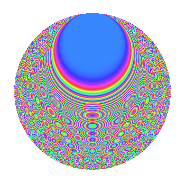# Properties

 Label 403.2.bsLevel 403 Weight 2 Character orbit bs Rep. character $$\chi_{403}(4,\cdot)$$ Character field $$\Q(\zeta_{30})$$ Dimension 288 Newforms 1 Sturm bound 74 Trace bound 0

# Related objects

## Defining parameters

 Level: $$N$$ = $$403 = 13 \cdot 31$$ Weight: $$k$$ = $$2$$ Character orbit: $$[\chi]$$ = 403.bs (of order $$30$$ and degree $$8$$) Character conductor: $$\operatorname{cond}(\chi)$$ = $$403$$ Character field: $$\Q(\zeta_{30})$$ Newforms: $$1$$ Sturm bound: $$74$$ Trace bound: $$0$$

## Dimensions

The following table gives the dimensions of various subspaces of $$M_{2}(403, [\chi])$$.

Total New Old
Modular forms 320 320 0
Cusp forms 288 288 0
Eisenstein series 32 32 0

## Trace form

 $$288q - 9q^{2} - q^{3} - 39q^{4} - 42q^{6} - 15q^{7} + 31q^{9} + O(q^{10})$$ $$288q - 9q^{2} - q^{3} - 39q^{4} - 42q^{6} - 15q^{7} + 31q^{9} + 3q^{10} - 18q^{11} - 46q^{12} - q^{13} - 32q^{14} + 18q^{15} + 21q^{16} - 15q^{17} - 15q^{19} + 51q^{20} - 10q^{22} - 4q^{23} - 51q^{24} - 296q^{25} - 6q^{26} - 52q^{27} + 21q^{28} + q^{29} + 60q^{30} + 138q^{32} + 69q^{33} - 10q^{35} + 128q^{36} - 18q^{37} + 32q^{38} - 14q^{39} + 60q^{40} - 15q^{41} - 49q^{42} - 36q^{43} + 6q^{45} - 69q^{46} + 21q^{48} - 23q^{49} + 117q^{50} + 8q^{51} + 26q^{52} - 48q^{53} + 75q^{54} + 46q^{55} - 98q^{56} - 21q^{58} - 105q^{59} - 74q^{61} - 3q^{62} - 90q^{63} + 90q^{64} + 89q^{65} - 8q^{66} + 6q^{67} - 182q^{68} + 29q^{69} + 3q^{71} - 183q^{72} - 53q^{74} - 38q^{75} + 144q^{76} - 128q^{78} - 72q^{79} - 72q^{80} + 11q^{81} - 11q^{82} - 33q^{84} + 72q^{85} - 18q^{87} - 14q^{88} + 81q^{89} - 34q^{90} - 48q^{91} + 8q^{92} + 72q^{93} - 6q^{94} + 141q^{97} + 96q^{98} + O(q^{100})$$

## Decomposition of $$S_{2}^{\mathrm{new}}(403, [\chi])$$ into irreducible Hecke orbits

Label Dim. $$A$$ Field CM Traces $q$-expansion
$$a_2$$ $$a_3$$ $$a_5$$ $$a_7$$
403.2.bs.a $$288$$ $$3.218$$ None $$-9$$ $$-1$$ $$0$$ $$-15$$